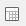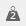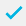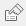# Changing the proportions (balance) of a sample group

The following example uses the Crocodile Rock Cafe Survey. In the survey there are more female respondents than male respondents. These samples can be balanced to represent half the respondents as female and half as male, without changing the current base.

1. Clickto display the Analysis Definition dialog for a table.
2. Specify the Analysis as Q12, which is the variable to be weighted. Build the table and note the totals for each code.
1. Calculate the Weighting Factors for each code.
• To calculate the value, divide the target figure (384÷2=192) by the actual figure.
• Code 1, Male = 192 ÷ 152 = 1.263
• Code 2, Female = 192 ÷ 232 = 0.828
2. Clickto display the Weights window.
3. Clickto add a new weight and specify the Weight Details as follows:

Name: WT1

Label: Weight male/female

Decimal places: 3

Number of codes: 2

1. Clickto save the weight.
2. Clickto redefine the table orto display a new Analysis Definition dialog.
3. Specify the Analysis as Q12, which is the variable to be weighted.
4. Specify the Weight as WT1 (Q12), which tells Snap to use weight WT1 based on the results for Q12.
5. Select the Cells tab. In the Accuracy section set the Calculations d.p. to 3 decimal places to avoid rounding errors.
6. Click OK to build the table. Check that the table shows the Weighted Values correctly, in this example there should be 103 males and 103 females. If errors are found this is either due to rounding errors (see step 7 above) or the Weighting Factors have been calculated incorrectly.

The table shows the Unweighted Base and the Weighted Base. The Unweighted Base can be excluded using the Tailor | Analysis option.

The weighting can be applied to any other tables and charts.

Multiple Response variables cannot be weighted.

You cannot use more than one weight in a table.

Contents